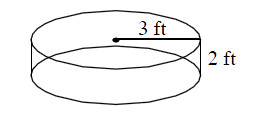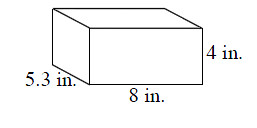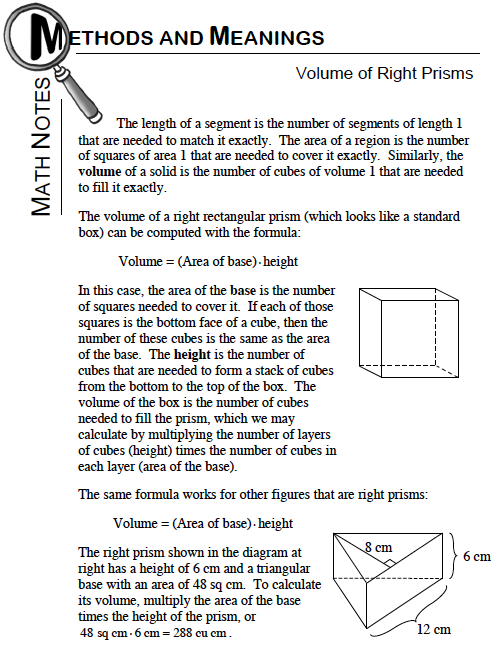### Home > MC1 > Chapter 10 > Lesson 10.1.1 > Problem10-12

10-12.

Find the volume of each of the following solids.

1. cylinder2. right rectangular prismFor parts (a) and (b), refer to the Math Notes box below.$\text{Remember, the area of a circle is } \frac{1}{2}\pi r^2.$

Also, remember that 'r' stands for radius, which is the distance from the center of the circle to a point on the outside of the circle.

To find the volume we will need to substitute the variables and simplify the expression.
If the base equals π(32) and the height is 2 feet, can you complete the calculations?

The volume of the cylinder is 18π cubic feet, or approximately 56.55 cubic feet.

Since the volume of a prism is the area of the base times the height, you should find the area of the rectangle first, then find the product of the area and the height. The area of a rectangle is the length times the width.